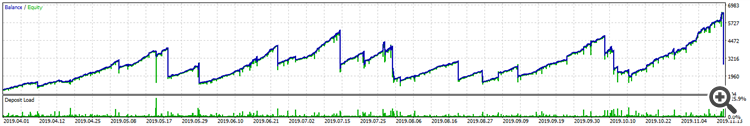# Hard SL

Hello, I'm having trouble with HardSL. It seems to be closing sometimes in a major percentage than I've set, these are the lines:

```void HardStop(){
double TotalProfit=0,TotalLoss=0;
double SymbolProfit=0,SymbolLoss=0;
for(int i=0;i<PositionsTotal();i++){
ulong iTicket=PositionGetTicket(i);
double Balance=AccountInfoDouble(ACCOUNT_BALANCE);
double PosLots=PositionGetDouble(POSITION_VOLUME);
double PosProfit=PositionGetDouble(POSITION_PROFIT);
if(PositionGetInteger(POSITION_MAGIC)==MagicNumber){
TotalProfit+=PosProfit;if(TotalProfit<0){TotalLoss=TotalProfit;}
if(PositionGetString(POSITION_SYMBOL)==_Symbol){
SymbolProfit+=PosProfit;if(SymbolProfit<0){SymbolLoss=SymbolProfit;}
}
}
if(HardStopLoss>0){ // Positive value sets HardSL true, I'm trying to close around 24%.
if(MathAbs(TotalLoss)>=HardStopLoss*Balance/100){
return;
}
else{i--;}
}
//break;
}
}
}```

Trying to close at 24% loss, I got this result (OHLC,M1):Falling below the 24% HardSL limit many times and finally got crashed... any idea on what's wrong? Thank you in advance.

Reason: